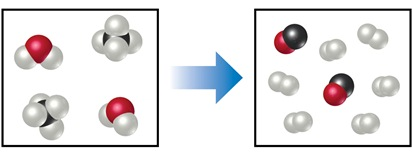# Problem: The diagram  represents a high-temperature reaction between CH4 and H2O.Based on this reaction, find how many moles of CO can be obtained starting with 6 mol CH4?

###### FREE Expert Solution

We’re asked to find how many moles of CO can be obtained starting with 6 mol CH4 for the given reaction based on the diagram.

To do so, we need to write a balanced chemical equation from the diagram.

The flow of the solution will be like this:

moles CH(mole-mole-comparison) → moles CO

First, we need to determine the reactants and products based on the diagram:

Reactants:

• 2 moles H2O (1 red and 2 white balls)
• 2 moles CH4 (1 black and 4 white balls)

Products:

• 2 moles CO (1 red and 1 black ball)
• 6 moles H2 (2 white balls)
91% (335 ratings)###### Problem Details

The diagramrepresents a high-temperature reaction between CH4 and H2O.

Based on this reaction, find how many moles of CO can be obtained starting with 6 mol CH4?

Frequently Asked Questions

What scientific concept do you need to know in order to solve this problem?

Our tutors have indicated that to solve this problem you will need to apply the Stoichiometry concept. You can view video lessons to learn Stoichiometry. Or if you need more Stoichiometry practice, you can also practice Stoichiometry practice problems.

What professor is this problem relevant for?

Based on our data, we think this problem is relevant for Professor Benedict's class at UB.